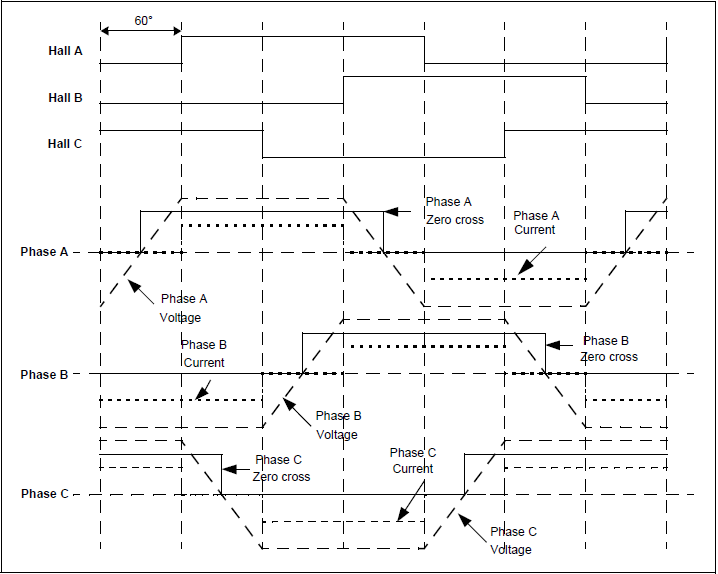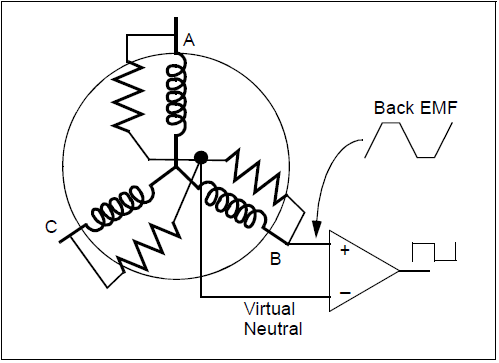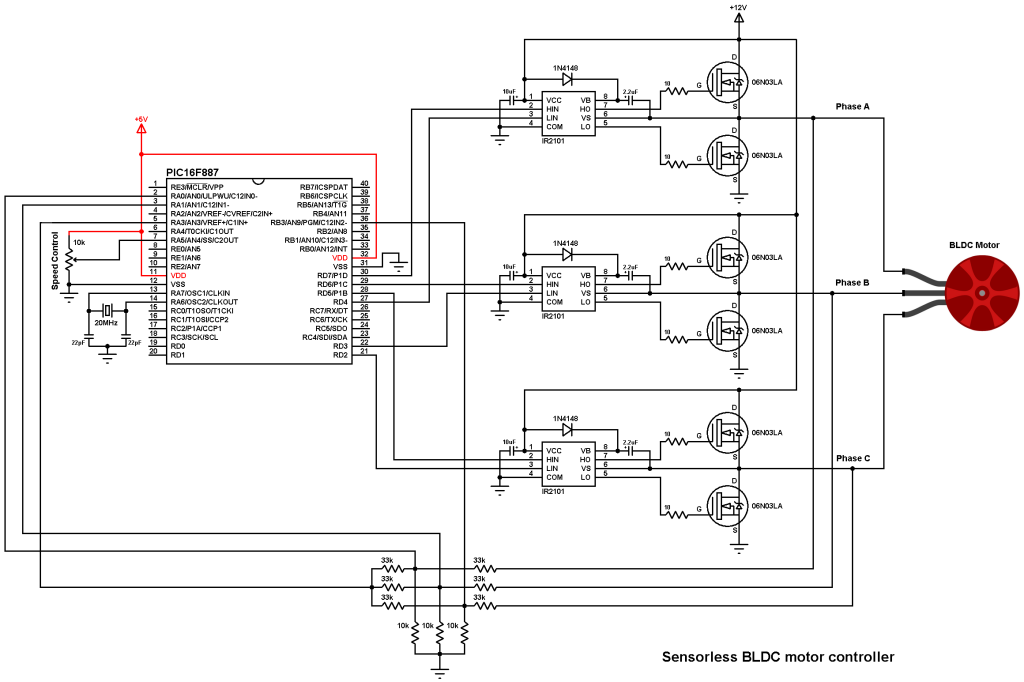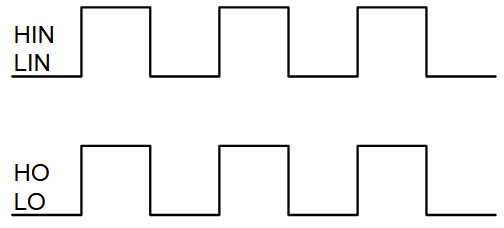# Brushless DC motor control with PIC16F887 microcontroller

This post shows how to control a sensorless brushless DC (BLDC) motor using PIC16F887 microcontroller, or let’s say how to build an ESC (Electronic speed controller using PIC16F887).

Basically there are two types of brushless motors: sensored and sensorless. Sensored BLDC motors come with three hall effect sensors which are used to detect (know) the position of the rotor (where it is located) at any moment, that’s why they called sensored.
The second type is: sensorless BLDC motors. Sensorless BLDC motor has no sensor to detect the rotor position, it has only 3 wires for power (3-phase). The commutation of sensorless BLDC motor is based on the BEMF (Back Electromotive Force) produced in the stator windings.

The main advantage of the sensorless BLDC motor control is lower system cost and the main disadvantage is the motor must be moving at minimum rate to produce sufficient BEMF to be sensed.

How it works:
When the BLDC motor rotates, each winding (3 windings) generates BEMF opposes the main voltage. The 3 generated BEMF signals are 120° out of phase which is the same as the hall effect sensor signals. The figure below shows the relationship between the hall effect signals and the BEMF signals:As shown in the figure above, the BEMF signals are not synchronized with the hall effect sensor signals (phase shift of 30°). In every energizing sequence, two windings are energized (one connected to positive and the other to negative) and the third winding is left open (floating). The floating winding is used to detect the zero crossing, thus, the combination of all 3 zero cross over point are used to generate the energizing sequence. Totally we’ve 6 events:
Phase A zero crossing: from high to low and from low to high
Phase B zero crossing: from high to low and from low to high
Phase C zero crossing: from high to low and from low to high

How to detect the zero crossing:
The easiest way to detect the zero crossing events is by using comparators. The comparator has 3 main terminals: 2 inputs (positive and negative) and an output. Comparator output is logic high if the positive voltage is greater than the negative voltage, and logic low if the positive voltage is lower than the negative voltage.
Basically 3 comparators are needed for this project, connections are done as shown in the figure below (example for phase B). Each phase requires a similar circuit:The virtual natural point is the same for all the 3 comparators, it is generated using 3 resistors. When the BEMF generated in the floating (open) winding crosses the zero point towards positive side, the comparator output makes a transition from low-to-high. When the BEMF generated in the floating winding crosses the zero point towards negative side, the comparator output makes a transition from high-to-low. By having three such comparator circuits, one on each of the phases gives three digital signals corresponding to the BEMF signal in the windings. The combination of these three signals is used to derive the commutation sequence.

The PIC16F887 has two analog comparators, one of them is enough for this project. The one will be used is comparator 1.

Components Required:

• PIC16F887 microcontroller – datasheet
• Brushless DC motor
• 6 x 06N03LA N-type mosfet (or equivalent)  – datasheet
• 3 x IR2101 (or IR2101S) gate driver IC  – datasheet
• 6 x 33k ohm resistor
• 3 x 10k ohm resistor
• 6 x 10 ohm resistor
• 3 x IN4148 diode
• 3 x 10uF capacitor
• 3 x 2.2uF capacitor
• 20 MHz crystal oscillator
• 2 x 22pF ceramic capacitor
• 10k ohm potentiometer
• 12V source
• 5V source
• Jumper wires

• CCS C compiler to compile the C code below
• PIC programmer such as PICkit 3, PICkit 2 ……
• MPLAB IPE software

Brushless DC motor control with PIC16F887 microcontroller circuit:
Project circuit schematic diagram is shown below.Note that all grounded terminals are connected together.

As known the brushless motors are 3-phase motors. In the circuit diagram above the 3 phases are named: Phase A, Phase B and Phase C.

In this project we need two power sources, the first one with 5V and used to power the microcontroller. The other one with 12V which is used to power the three-phase bridge and therefore the BLDC motor.

The first three 33k (connected to motor phases) and the three 10k resistors are used as voltage dividers, because we can not supply the microcontroller with 12V, the other three 33k resistors generate the virtual natural point. The virtual natural point is connected to pin RA3 (#5) which is comparator 1 positive input.

PIC16F887 microcontroller has two analog comparators, one of them is enough for this project, so I chose comparator 1. The positive input of this comparator is C1IN+ (pin #5) and the negative input can be C12IN0- (pin #2), C12IN1- (pin #3), C12IN2- (pin #36) or C12IN3- (pin #34). Therefore, I connected the virtual natural point to the positive pin of the analog comparator C1IN+ (pin #5), phase A BEMF to C12IN0- (pin #2), C12IN1- (pin #3) and phase C BEMF to C12IN2- (pin #36). Each time the comparator compares the virtual point with the BEMF of one phase (done in the software). This minimizes the hardware needed and simplifies the circuit.

The IR2101 chips are used to control high side and low side mosfets of each phase. The switching between the high side and the low side is done according to the control lines HIN and LIN. The figure below shows input and output timing diagram:The HIN lines of the three IR2101 are connected to pins: P1D (RD7), P1C (RD6) and P1B (RD5) respectively for phase A, phase B and phase C, whereas the LIN lines are connected to pins: RD4, RD3 and RD2.
The PIC16F887 has one CCP module (Capture/Compare/PWM module) and one ECCP module (Enhanced CCP module). With the ECCP module we can generate 4 PWM signals with the same frequency and controlled by one duty cycle (we need 3 PWM signals). In this project I applied 3 PWM signals to high side mosfets only (one PWM active at a time).

The speed of the BLDC motor is controlled by a potentiometer connected to analog channel AN4 (pin #7).

The PIC16F887 runs with 20MHz crystal oscillator (5 MIPS), MCLR pin function is disabled.

Brushless DC motor control with PIC16F887 microcontroller C code:
The C code was tested with CCS C compiler version 5.051. The compiler may give a warning, it is not a problem at all, just ignore it!

Timer2 module is configured to give a PWM signal with frequency of 19.53 KHz and resolution of 10 bits. The configuration line is:
setup_timer_2(T2_DIV_BY_1, 255, 1);
where Timer2 prescaler = 1, preload (PR2) = 255
PWM frequency and resolution can be calculated using the functions below (Fosc = 20 MHz):
PWM_freqency = Fosc/{[(PR2) + 1] * 4 * (TMR2 Prescaler Value)} = 19.53 KHz
Resolution = log[4(PR2 + 1)]/log(2) = 10 bits

Full CCS C code is below.

Finally this video shows a simple hardware circuit of the project:

References:
Microchip AN970 document
https://www.microchip.com/

1.Toto says:

Do you have an idea if we would like to do the direction change.

2.Son says:

I cant load C code on PIC 16f887, Please send me file .hex of project

1.Iván says:

X2… I can’t compile this C code. I need .hex

3.Asen says:

1.Simple Projects says: